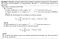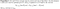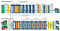# Generative Adversarial Network and Super Resolution GAN(SRGAN)

Image super-resolution (SR) techniques reconstruct a higher-resolution image from the observed lower-resolution images. An intuitive method for this topic is interpolation, for which texture detail in the reconstructed images is typically absent.

Super-Resolution Generative Adversarial Network, or SRGAN, is a generative adversarial network (GAN) for image super-resolution that is more appealing to human perspective.

# Brief review of GAN

GAN is comprised of two neural networks, Generator and Discriminator. GAN learns a probability distribution of a dataset by pitting these two neural networks against each other.

The structure is shown in below:

Notation

Discriminator

Goal: discriminate whether the data were real or not.
input: half real data + half generated from generator (fake data)
loss function:

Generator

Goal: create data that look very similar to the real dataset to fool discriminator. It used the prediction of discriminator to feedback and update model.
input: noise data
loss function:

The optimization procedure is that fix G to update parameters of D(base on loss of discriminator) then fix D to update parameters of G(base on loss of generator).GAN algorithm

This entire process is just like playing the following two-player minimax game with value function V(G,D).

When generator can successfully fool discriminator, we can treat this generator as a distribution transformer to simulate the real data distribution.

# Generate random variable

When we talk about generating random variable from any specific distribution, the standard method are inverse transform method, Accept-Reject sampling or Metropolis-Hasting… MCMC algorithms. However, if I want to generate image of dog from dog distribution, this is difficult to apply the traditional method mentioned above.

What is “probability distributions for images of dogs” means? This is an abstract idea. It’s hard to write down the density function of image.

GAN treats neural network as a powerful black box that can learn directly from data how to generate data from any empirical distribution.

Given a density function which we can easily sample data from. We can use trained generator network in GAN to transform data into the other specific complicated data distribution. The original GAN paper also have proven that “if given enough capacity and training time, p_g will converge to p_data”.https://arxiv.org/pdf/1406.2661.pdf

For a simple example, assume that I can only generate data from uniform distribution and I have some observed data. I don’t know what is the ground-true distribution of observed data but I want to sample more data from the distribution behind the observed data(here we assume it’s normal distribution).

The idea is that training a generator network by GAN to transform uniform distribution(which we can generate) to empirical distribution of observed data(whose ground-true distribution is normal).

Refer to keras -GAN, I use only one fully connected layer with 50 neurons in generator network and two fully connected layers with 128 neurons in discriminator network.

Sample 1000 data from normal distribution as our observed data, then train 30000 epochs. Let’s see some generator output during training:

We can see that the data generated from generator is more and more like normal shape.

# Anomaly detection

Generator also can be applied to do anomaly detection.
Assume that in common situation, we have data from uniform distribution and generator had learned how to transform uniform data to normal distribution. So, when generator outputs data which are not follow normal distribution, we can suspect that the original data may not follow uniform distribution anymore.

`import numpy as npfrom keras.models import load_modelimport matplotlib.pyplot as pltfrom scipy import statsgenerator_path = './models/generator_model_30000.hdf5'sample_size = 100generator =  load_model(generator_path)noise = np.random.uniform(0, 1, (sample_size, 1))#sample one dimensional uniformbatch_samples = generator.predict(noise)samples = [i for i in batch_samples]statistic,p_value = stats.kstest(samples, 'norm',args=(0,1))plt.hist(noise,density=True)plt.show()plt.hist(samples,density=True)plt.title('p value is %.3f'%p_value)plt.show()`

We can see that the p-value of ks-test is 0.131 which is larger than 0.05. We can not reject null hypothesis and conclude that the data after transformed by generator are follow normal distribution.

Support that our data source suffer some issue such that data don’t follow uniform distribution anymore(e.g. beta distribution).

`## test other distributionnoise = np.random.beta(1,5,sample_size)batch_samples = generator.predict(noise)samples = [i for i in batch_samples]statistic,p_value = stats.kstest(samples, 'norm',args=(0,1))plt.hist(noise)plt.show()plt.hist(samples)plt.title('p value is %.3f'%p_value)plt.show()`

We can see that generator failed to transform data to normal distribution (p-value=0, reject H0) which implied that there are some problem from data source.

# Super Resolution GAN (SRGAN)

With basic knowledge of GAN, we might image that how can we generate high resolution image(HR) from low resolution image(LR).

Let the real data of GAN be HR image, and noise data be LR image, then let generator to learn how to generate super resolution image(transform LR image to HR) through GAN training procedure. If everything goes well, fake HR will eventually converge to HR. That is!

The perceptual loss of SRGAN for the generator composes of content loss and adversarial loss.

Content loss:

Extract feature map of HR image and fake HR image from VGG-19 and compute the MSE between these two features.The pixel-wise MSE loss is calculated as: (MSE between two feature map)

Where φi,j indicate the feature map obtained by the j-th convolution (after activation) before the i-th maxpooling layer within the VGG19 network.

Adversarial loss:

Same as normal GAN generator loss

For each batch update:

1. Sample half batch of HR(real data) and downsampling to LR.
2. Sample another half batch of HR then feed it into generator network to get fake HR.
3. Feed HR and fake HR to discriminator and use typical GAN discriminator loss to update discriminator network
4. Feed LR into generator+discriminator(fixed) and use perceptual loss which described above to update generator network.https://arxiv.org/pdf/1609.04802.pdf

There are awesome GAN github we can apply(base on keras and pytorch)

I downloaded CelebFaces data from here and train SRGAN with 30,000 epochs. Set LR resolution as 60*60 and HR resolution 240*240(4*upscaling).

I randomly took some images from internet to test the Generator network:

It looks not bad, right? Nevertheless, I found that generator performs badly on eyes area especially when dealing with people with thick glasses.
It may due to this kind of image is less in training data.

# Reference

Generative Adversarial Networks

Photo-Realistic Single Image Super-Resolution Using a Generative Adversarial Networ

Understanding Generative Adversarial Networks (GAN)

GAN — Super Resolution GAN (SRGAN)